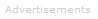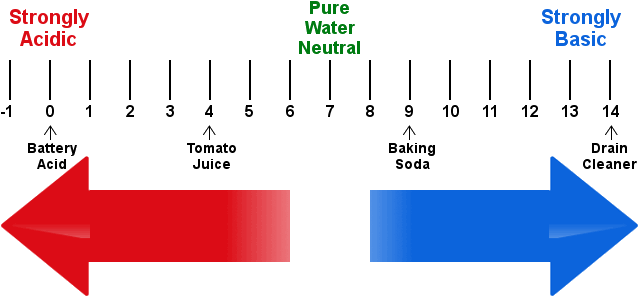# Definition of pH

What is pH?

The pH scale is alternatively sometimes called the pH-acid-base scale and sometimes just the acid-base scale.In neutral solutions, i.e. those that are neither acidic nor basic, pH = 7.0.

Acidic solutions are those with pH less than 7, while basic solutions have pH greater than 7.

A solution is:

• acidic when there are more H+ ions than OH- ions present
• basic when there are more OH- ions than H+ ions present
• neutral when there are equal number of H+ and OH- ions present

pH is a measure of the hydrogen ion activity, typically in aqueous solution.

In pH, the p stands for -log10 and the H stands for hydrogen ion activity:

pH = -log10aH+

In dilute solutions, the activity of hydrogen ions can be approximated by their molar concentration, and it is in this form that pH is normally used:

pH = -log10[H+]
Why is pH=7 Neutral in Aqueous Solutions?

To see why this should be so, consider the following:

Water exists as an equilibrium between molecules and ions:

H2O ⇌ H+ + OH-

The multiplication product of the concentrations [H+] and [OH-] is a constant; it is water's dissociation constant, which has a known value of 10-14 M2.

Kw = [H+][OH-] = 10-14 M2

For neutrality, [H+] must be equal to [OH-]. This means these quantities must both equal 10-7 M.

If we put [H+] = 10-7 M into the equation for pH:

pH = -log10[H+]

we get pH = 7 for neutrality.

Acid and Base pH

Acid solutions have pH < 7. pH values lower than 0 are possible.

Basic solutions have pH > 7. pH values greater than 14 are possible.The pH ScaleSearch the Dictionary for More Terms